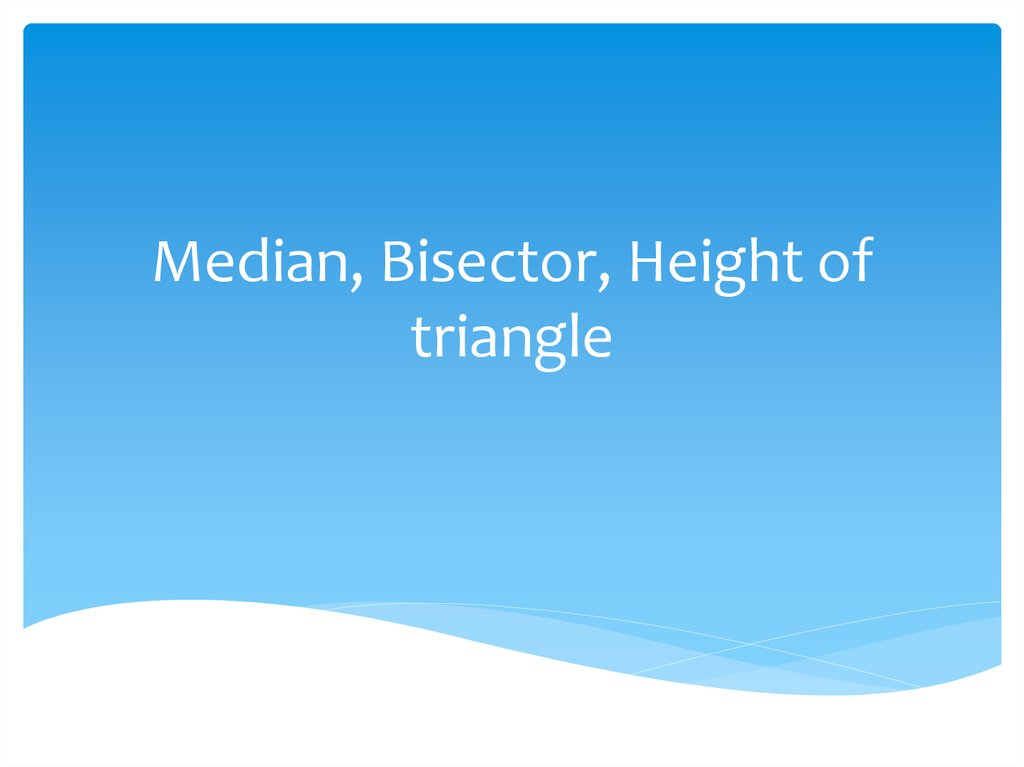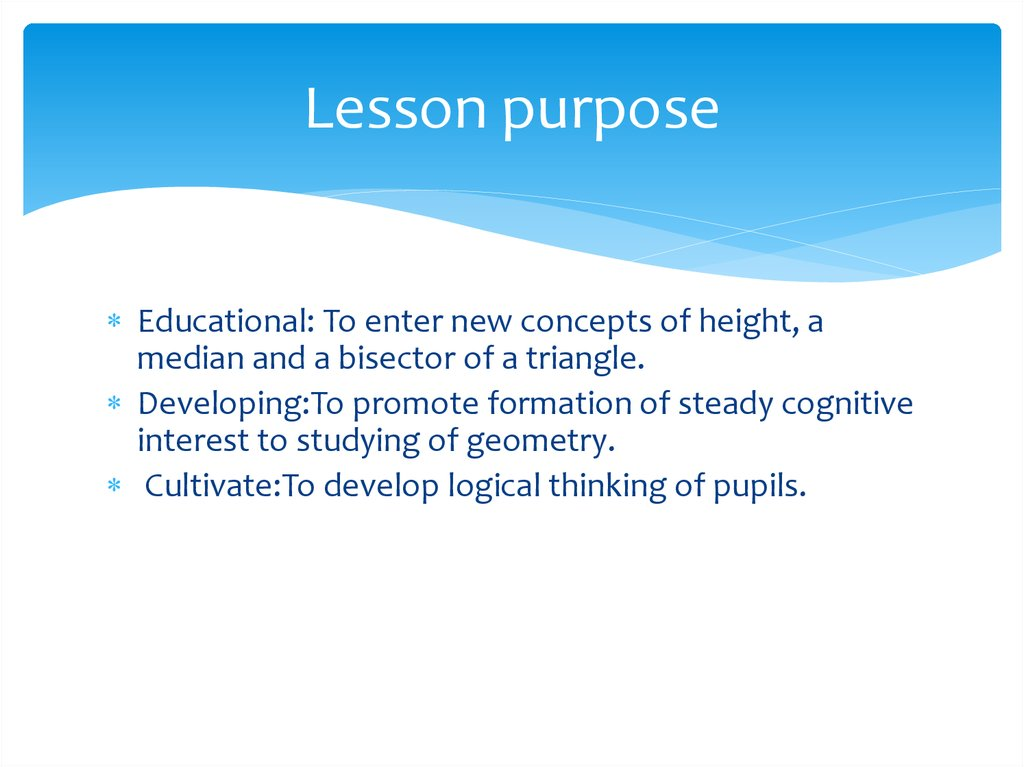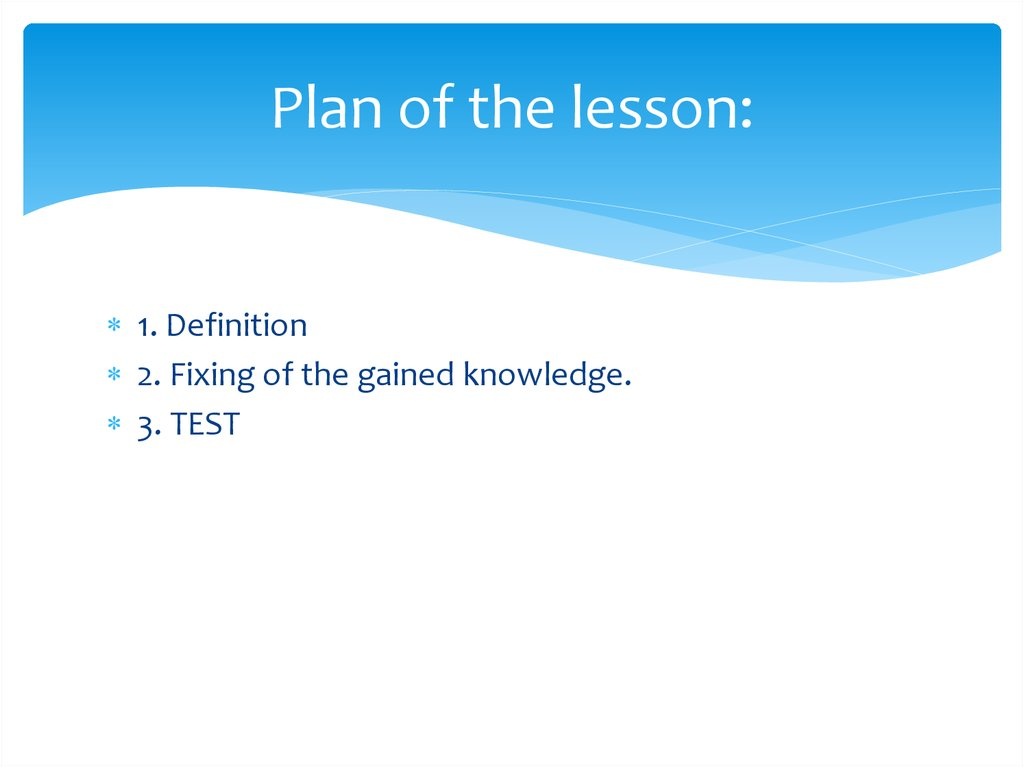Median, Bisector, Height of triangle

2. Lesson purpose

Educational: To enter new concepts of height, a
median and a bisector of a triangle.
Developing:To promote formation of steady cognitive
interest to studying of geometry.
Cultivate:To develop logical thinking of pupils.

3. Plan of the lesson:

1. Definition
2. Fixing of the gained knowledge.
3. TEST

4. 1. Bisector

The bisector is a beam which proceeds from his top,
passes between his parties and halves this corner. A
bisector of a triangle is called the triangle bisector
piece connecting top to a point on the opposite party
of this triangle.

6. Questions {Bisector}

• Formulate property of bisectors of a triangle.
(In any triangle of a bisector are crossed in one point).

7. Median

The median is the piece connecting triangle top to the
middle of the opposite side.

9. 2. Questions {Median}

• How many medians it is possible to carry out in a
triangle? (3).
• "Carry out" three medians in a triangle.
• What property of medians you have noticed? (In
any triangle all medians are crossed in one point).

10. Height

Height is the perpendicular which is carried out from
triangle top to the straight line containing the
opposite side.

12. Questions {Height}

• "Construct" all three heights in a triangle.
• Whether heights similar property, as medians have?
(Yes).
• And if a triangle obtusangular, then how to
construct heights? (To carry out additional half-lines)
• How you think what is height in a rectangular
triangle?

13. 3. TEST

1. Fill in admissions in formulations of elements of a triangle and properties of
geometrical figures.
a) The piece connecting triangle top to the middle ______________ is called
___________ a triangle.
(The piece connecting triangle top to the middle of the opposite side is called a
triangle median).
b) From the point which isn't lying on a straight line it is possible to carry out a
perpendicular to this straight line, and besides _____________.
(From the point which isn't lying on a straight line it is possible to carry out a
perpendicular to this straight line, and besides only).
2. Whether Verna following statements?
a) In any triangle it is possible to carry out three medians. (Yes).
b) The point of intersection of heights of any triangle lies in a triangle. (Not always).
c) All bisectors of a triangle are crossed in one point. (Yes).